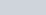ChemTalk

# Calculating Percent Yield## Core Concepts

In this tutorial, you will learn calculating percent yield and theoretical yield are, and how to calculate it. In addition, you will walk through an example calculation.

## What is Percent Yield?

When performing an experiment, there is a maximum yield you can obtain if there are perfect reaction conditions; this is the theoretical yield. However, even if you follow an experiment correctly, it is likely that you will not have a perfect yield of the product; the amount of product you end up with is your actual yield.

The percentage of the theoretical yield you obtained in your experiment is the percent yield. Let’s learn how to calculate it below!

## How to Calculate Percent Yield

You can use the equation below to calculate your yield from an experiment: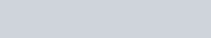## What is Theoretical Yield

The theoretical yield is the maximum amount of product that can be obtained from a chemical reaction. It is calculated based on the stoichiometry of the reaction, which is the study of the proportions of the reactants and products in a chemical reaction. The theoretical yield is determined by using the balanced chemical equation for the reaction and the known amounts of the reactants.

For example, if you have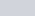of reactant A and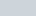of reactant B, and the balanced chemical equation for the reaction is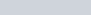, then the theoretical yield of product C would be, because that is the maximum amount that could be produced from the given amount of reactants. In reality, however, the actual yield of a reaction is often less than the theoretical yield, due to a variety of factors such as incomplete conversion of the reactants or loss of product during the reaction.

### Calculating Theoretical Yield

First, you should calculate the theoretical yield of your experiment; usually, this will involve stoichiometric calculations. By first looking at the chemical equation and information given, you can get an idea of what is reacting and how the product is forming.

Since no more product can be formed once the limiting reagent runs out, the next step is to identify the limiting reagent.

You can then use dimensional analysis to see how much product can be formed based on the amount of limiting reagent that is given. This is the theoretical yield of your experiment.

### Calculating Actual Yield

If you are physically doing an experiment, your actual yield will be the amount of product you weigh out on your balance. If you are doing a word problem, the actual yield may be given within the problem.

### Percent Yield Equation

The last step, once you have both theoretical and actual yield, is plugging the numbers into the equation. Dividing the actual by the theoretical gives you the fraction of product you made. Multiplying that by 100 gives you the percent yield.

## Calculating Percent Yield Example

Now that we know the steps to calculate yield, let’s walk through an example:

Use the balanced chemical reaction below. If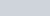of Acetylene (C2H2) and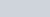of Oxygen are used, and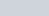of water are produced, what is the percent yield?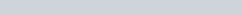First step is to find limiting reagent & theoretical yield of water:Using dimensional analysis on both reagents, water is found to produce a lower amount of product than oxygen; because of this water is our limiting reagent.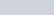is our theoretical yield. The last step is to plug our numbers into the percent yield equation.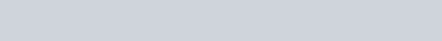Our yield is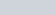.

## Calculating Percent Yield Practice Problems

Problem 1

You mix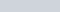of MgCl2 and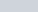of AgNO3 and recover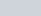of AgCl. Calculate the yield.

MgCl2 and AgNO3 react according to the following equation: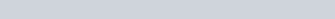Problem 2

You drop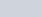of elemental sodium (Na) into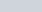of water and collect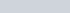of H2. Calculate the percent yield. Sodium and water react according to the following reaction: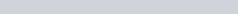## Calculating Percent Yield Practice Problem Solutions

1: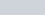2: